# Decimals Worksheets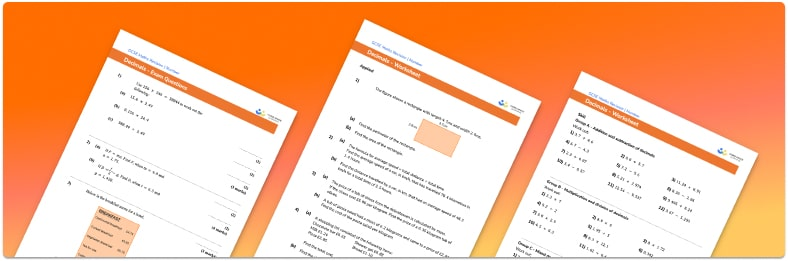• This field is for validation purposes and should be left unchanged.

You can unsubscribe at any time (each email we send will contain an easy way to unsubscribe). To find out more about how we use your data, see our privacy policy.

• Section 1 of the decimals worksheet contains 36 skills-based decimals questions, in 3 groups to support differentiation
• Section 2 contains 4 applied ​​decimals questions with a mix of word problems and deeper problem solving questions
• Section 3 contains 4 foundation and higher level GCSE exam style decimals questions
• Answers and a mark scheme for all decimals questions are provided
• Questions follow variation theory with plenty of opportunities for students to work independently at their own level
• All division problems created by fully qualified expert secondary maths teachers
• Suitable for GCSE maths revision for AQA, OCR and Edexcel exam boards

### Decimals at a glance

If a number has a part that is not whole, it is called a decimal number. We write the whole number part, followed by a decimal point, then tenths, hundredths, thousandths, and so on. It is important that students can recognise the decimal place value of each column on a place value chart – for example, know that 0.4 represents 4 tenths. Ordering decimals also requires students to apply this knowledge of place value.

Decimal arithmetic is similar to whole number arithmetic. Decimal addition follows the same method as integer addition, as does decimal subtraction. When multiplying decimals, we use powers of ten to convert our calculation into a whole-number multiplication, then reverse this process to get our decimal answer.

There are a couple of different methods for decimal division. If it is just the dividend that is a decimal, with an integer divisor, the standard algorithm for short or long division can be used. We have to ensure the decimal point is placed in the quotient (answer) directly above its position in the dividend.

If the divisor is a decimal, we write the division as a fraction and use the concept of equivalent fractions to convert to a whole-number calculation.

Looking forward, students can then progress to additional number worksheets, for example a percent worksheet, a rounding decimals worksheet or anFor more teaching and learning support on Number our GCSE maths lessons provide step by step support for all GCSE maths concepts.

## Related worksheets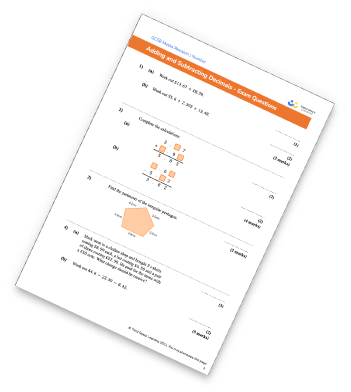Multiplying And Dividing Decimals Worksheet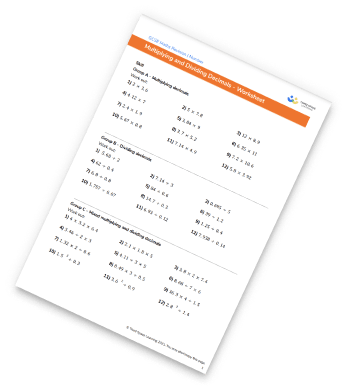Multiplying Fractions Worksheet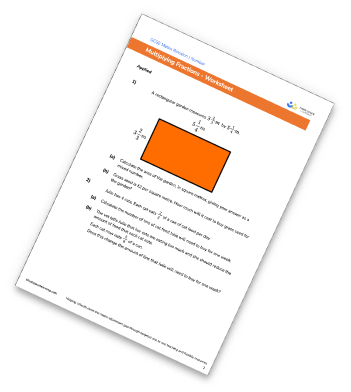Rational And Irrational Numbers Worksheet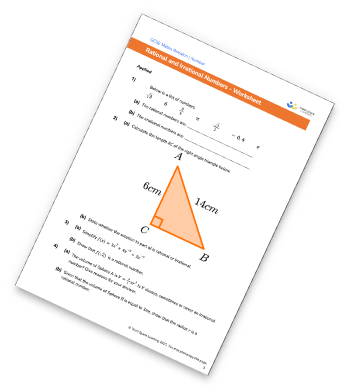## Do you have KS4 students who need more focused attention to succeed at GCSE?There will be students in your class who require individual attention to help them succeed in their maths GCSEs. In a class of 30, it’s not always easy to provide.

Help your students feel confident with exam-style questions and the strategies they’ll need to answer them correctly with our dedicated GCSE maths revision programme.

Lessons are selected to provide support where each student needs it most, and specially-trained GCSE maths tutors adapt the pitch and pace of each lesson. This ensures a personalised revision programme that raises grades and boosts confidence.

Find out more